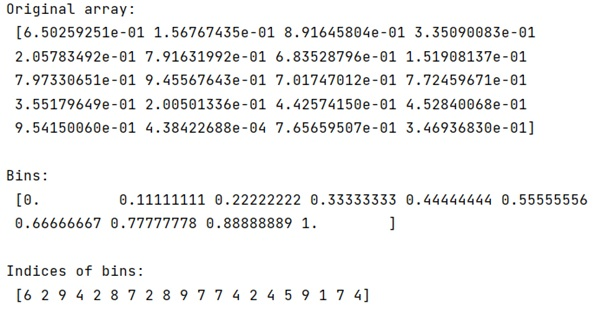# Python | Get Indices of Histogram Bins

Get Indices of Histogram Bins: In this tutorial, we will learn how to efficiently get the indices of histogram bins in Python? By Pranit Sharma Last updated : April 14, 2023

## What are Histogram Bins?

A histogram is an approximate representation of the distribution of numerical data. The representation is done by grouping the data into bins. Each bin is plotted as a bar whose height corresponds to how many data points are in that bin.

## How to Get Indices of Histogram Bins?

To get indices of histogram bins, we can simply use the numpy.digitize() method which is used to return the indices of the bins to which each value in the input array belongs.

The concept of bins in digitize() method is like a range between which a value of a numpy array may lie. There is a parameter in digitize() method called right which means in a certain range, we want the higher value to be considered or not.

Suppose that we are having a bin array as [1, 2, 3, 4] and an input array as [1.5], now since 1.5 lies between 1 and 2 and if we do not consider the higher values of the range 1 and 2, it will return the index of 1 that is 0.

Let us understand with the help of an example,

## Python Program to Get Indices of Histogram Bins

```# Import numpy
import numpy as np

# Creating a data set
vals = np.random.random(20)

# Display original array
print("Original array:\n",vals,"\n")

# Creating bins
bins = np.linspace(0, 1, 10)

# Display bins
print("Bins:\n",bins,"\n")

# Getting indices of bins
ind = np.digitize(vals, bins)

# Display result
print("Indices of bins:\n",ind)
```

### Output